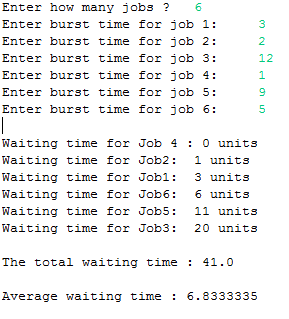## Thursday, 15 January 2015

### CPU Scheduling algorithm - SJF (Java)

 This is a Java program which implements one of the CPU Scheduling algorithm called Shortest Job First(SJF). Shortest Job First algorithm reduces the waiting time  but it is impossible to implement  as the processor must know all the jobs/processes  in advance.
PROGRAM :
```package codingcorner.in;

import java.util.Scanner;

public class SJF {
public static void main(String[] args) {
int i, j, temp, temp2, n;
float avgWt, totalWt = 0;
int a[] = new int;
Scanner scan = new Scanner(System.in);

System.out.print("Enter how many jobs ?\t");
n = scan.nextInt();
int bt[] = new int;
int wt[] = new int;

wt = 0;
for (i = 1; i <= n; i++) {
System.out.print("Enter burst time for job " + i);
bt[i] = scan.nextInt();
a[i] = i; // stores job has how much burst time in array i
}
scan.close();
for (i = 1; i <= n; i++)
// ascending order of burst times and a[i]

for (j = i; j <= n; j++)

if (bt[i] > bt[j]) {
temp = bt[i];
bt[i] = bt[j];
bt[j] = temp;
temp2 = a[i];
a[i] = a[j];
a[j] = temp2;
}

System.out.print("\nWaiting time for Job " + a[i] + " : 0 units \t");
for (i = 2; i <= n; i++) {
wt[i] = bt[i - 1] + wt[i - 1];
System.out.print("\nWaiting time for Job" + a[i] + ":\t" + wt[i]
+ " units \t");
totalWt = totalWt + wt[i];
}
System.out.print("\n\nThe total waiting time : " + totalWt);
avgWt = totalWt / n;
System.out.println("\n\nAverage waiting time : " + avgWt);
}
}
```

OUTPUT :Java - CPU Scheduling algorithm(SJF)